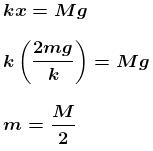Courses

# Work, Power And Energy MCQ Level - 1

## 10 Questions MCQ Test Topic wise Tests for IIT JAM Physics | Work, Power And Energy MCQ Level - 1

Description
This mock test of Work, Power And Energy MCQ Level - 1 for IIT JAM helps you for every IIT JAM entrance exam. This contains 10 Multiple Choice Questions for IIT JAM Work, Power And Energy MCQ Level - 1 (mcq) to study with solutions a complete question bank. The solved questions answers in this Work, Power And Energy MCQ Level - 1 quiz give you a good mix of easy questions and tough questions. IIT JAM students definitely take this Work, Power And Energy MCQ Level - 1 exercise for a better result in the exam. You can find other Work, Power And Energy MCQ Level - 1 extra questions, long questions & short questions for IIT JAM on EduRev as well by searching above.
QUESTION: 1

### A ring of mass m can slide over a smooth vertical rod. The ring is connected to a spring of force constant k = 4mg/R  where 2R is the natural length of the spring. The other end of the spring is fixed to the ground at a horizontal distance 2R  from the base of the rod. The mass is released at a height of 1.5R from the ground.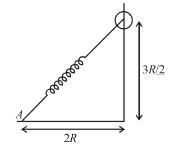Solution: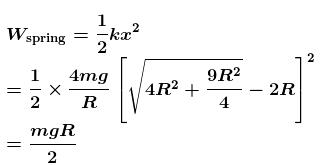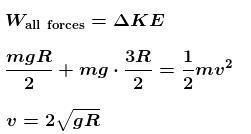The correct answer is: the velocity of the ring when it reaches the ground will be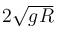QUESTION: 2

### A body is dropped from a certain height. When it loses U amount of its energy it acquires a velocity v. The mass of the body is :

Solution: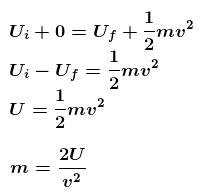The correct answer is: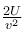QUESTION: 3

### A body is moved along a straight line by a machine delivering a constant power. The distance moved by the body in time t is proportional to :

Solution: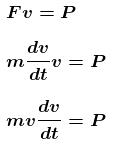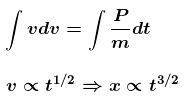QUESTION: 4

A self propelled vehicle of mass m whose engine delivers constant power P has an acceleration a = P/mv (assume that there is no friction). In order to increase its velocity from v1 to v2 the distance it has to travel will be :

Solution: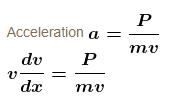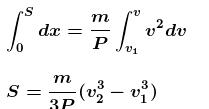The correct answer is: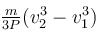QUESTION: 5

A block attached to a spring, pulled by a constant horizontal force, is kept on a smooth surface as shown in the figure. Initially, the spring is in the natural state. Then the maximum positive work that the applied force F can do is :

[Given that spring does not break]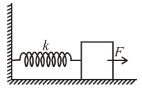Solution:

Applying work-energy theorem on the block, we get
Fl − kt2/2 = 0
l = 2F/k
or work done = Fl = 2F2/k

QUESTION: 6

A block of mass m is attached to two unstretched springs of spring constants k1 and k2 as shown in figure. The block is displaced towards right through a distance x and is released. Find the speed of the block as passes through the means position shown.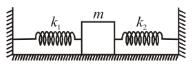Solution: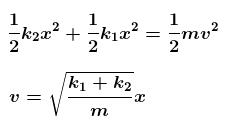The correct answer is: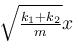QUESTION: 7

The block of mass m initially at x = 0 is acted upon by a horizontal force at any position x is given as F = a  bx2, where a > μmg, as shown in the figure. The co-efficient of friction between the surfaces of contact is μ. The net work done on the blocks is zero. If the block travels a distance?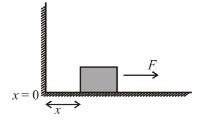Solution: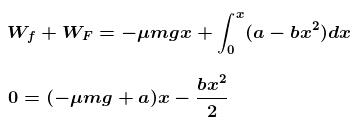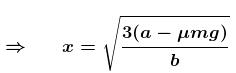The correct answer is: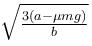QUESTION: 8

A man places a chain (of mass m and length l) on a table slowly. Initially the lower end of the chain just touches the table. The man drops the chain. When half of the chain is in vertical position. The work done by the man in this process is :

Solution:

The work done by man is negative of magnitude of decrease in potential energy of chain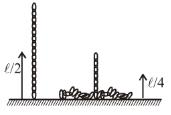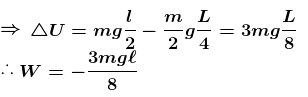The correct answer is: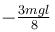QUESTION: 9

A body is projected with kinetic energy k at angle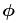with the vertical. Neglecting friction, its potential energy at the highest point will be :

Solution:

KE + PE = k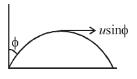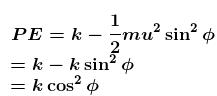The correct answer is: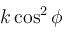QUESTION: 10

In the Figure, the ball A is released from rest when the spring is at its natural length. For the block B of mass M to leave contact with the ground of some stage, the minimum mass of A must be :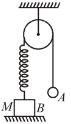Solution:

Let m be minimum mass of ball
Let mass A moves downwards by x.
From conservation of energy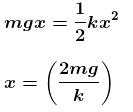For mass  to leave contact with ground,